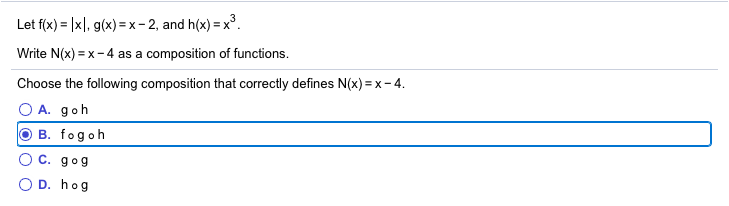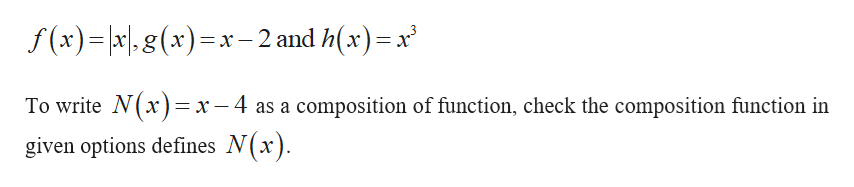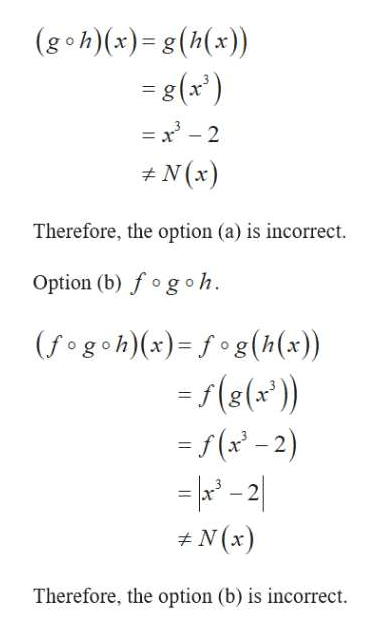# Let f(x) = |x|, g(x) = x - 2, and h(x) =x°.Write N(x) = x-4 as a composition of functions.Choose the following composition that correctly defines N(x) = x- 4.O A. gohO B. fogohC. gogD. hog

Question
52 viewshelp_outlineImage TranscriptioncloseLet f(x) = |x|, g(x) = x - 2, and h(x) =x°. Write N(x) = x-4 as a composition of functions. Choose the following composition that correctly defines N(x) = x- 4. O A. goh O B. fogoh C. gog D. hog fullscreen
check_circle

Step 1

The given functions are,help_outlineImage Transcriptionclosef (x)=|x|,g(x)=x- 2 and h(x)=x To write N(x)= x - 4 as a composition of function, check the composition function in given options defines N(x). fullscreen
Step 2

Option (a...help_outlineImage Transcriptionclose(g•h)(x)= g(h(x)) %3D = g(x') = x' - 2 %3D + N (x) Therefore, the option (a) is incorrect. Option (b) fogoh. (fogoh)(x)= f°g(h(x)) = f(8(x')) = f (x' - 2) = x* - 2| %3D + N (x) Therefore, the option (b) is incorrect. fullscreen

### Want to see the full answer?

See Solution

#### Want to see this answer and more?

Solutions are written by subject experts who are available 24/7. Questions are typically answered within 1 hour.*

See Solution
*Response times may vary by subject and question.
Tagged in
MathCalculus

### Other